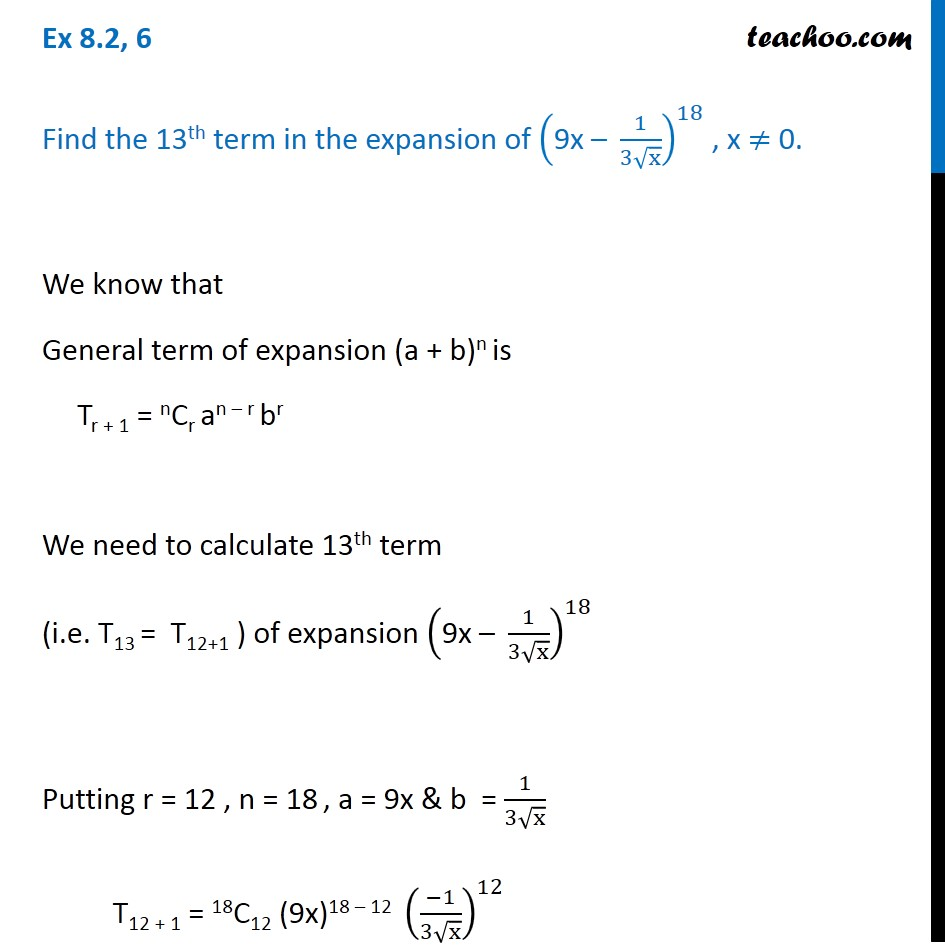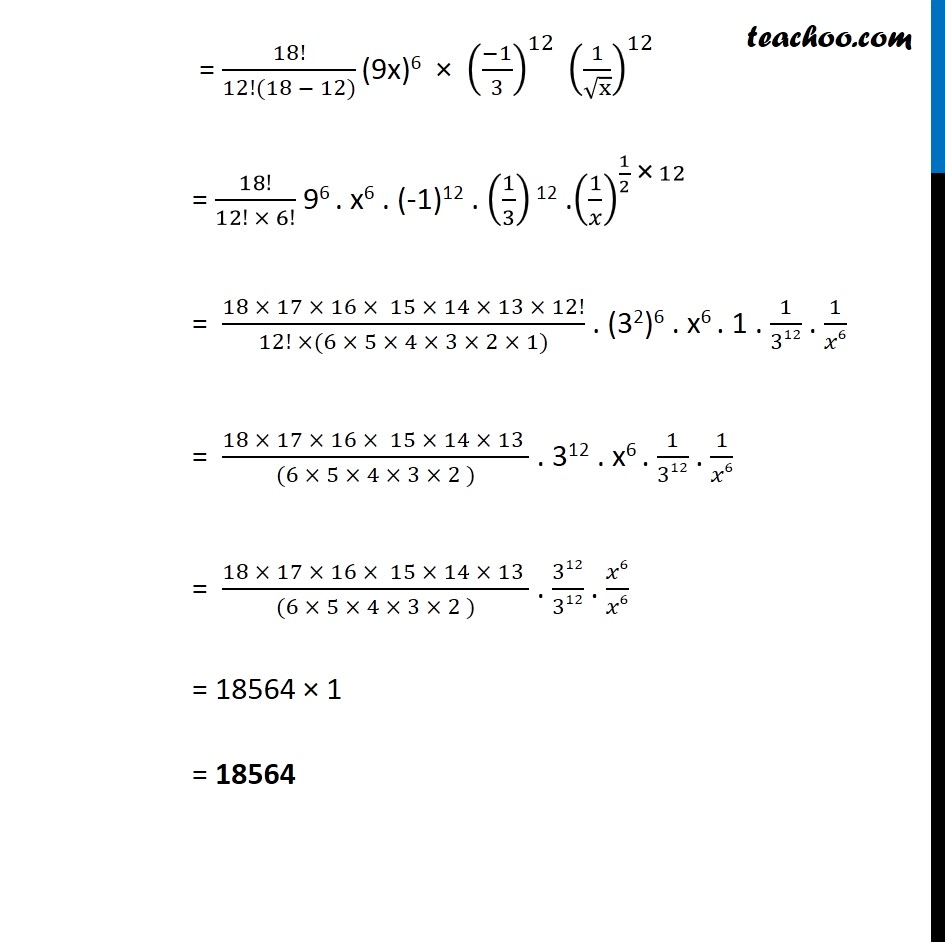General Term of Binomial Theorem

Chapter 7 Class 11 Binomial Theorem
Serial order wiseLearn in your speed, with individual attention - Teachoo Maths 1-on-1 Class

### Transcript

Question 6 Find the 13th term in the expansion of ("9x – " 1/(3√x))^18 , x ≠ 0. We know that General term of expansion (a + b)n is Tr + 1 = nCr an – r br We need to calculate 13th term (i.e. T13 = T12+1 ) of expansion ("9x – " 1/(3√x))^18 Putting r = 12 , n = 18 , a = 9x & b = 1/(3√x) T12 + 1 = 18C12 (9x)18 – 12 ((−1)/(3√x))^12 = 18!/(12!(18 − 12)) (9x)6 × ((−1)/3)^12 (1/√x)^12 = 18!/(12! × 6!) 96 . x6 . (-1)12 . (1/3) 12 .(1/𝑥)^(1/2 " ×" 12) = (18 × 17 × 16 × 15 × 14 × 13 × 12!)/(12! ×(6 × 5 × 4 × 3 × 2 × 1)) . (32)6 . x6 . 1 . 1/312 . 1/𝑥6 = (18 × 17 × 16 × 15 × 14 × 13 )/((6 × 5 × 4 × 3 × 2 )) . 312 . x6 . 1/312 . 1/𝑥6 = (18 × 17 × 16 × 15 × 14 × 13 )/((6 × 5 × 4 × 3 × 2 )) . 312/312 . 𝑥6/𝑥6 = 18564 × 1 = 18564